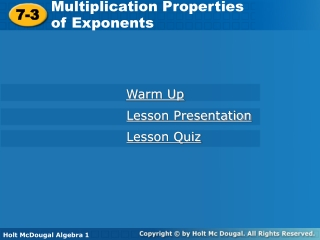Download Presentation7-3

# 7-3

Télécharger la présentation## 7-3

- - - - - - - - - - - - - - - - - - - - - - - - - - - E N D - - - - - - - - - - - - - - - - - - - - - - - - - - -
##### Presentation Transcript

1. Multiplication Properties of Exponents 7-3 Warm Up Lesson Presentation Lesson Quiz Holt McDougal Algebra 1 Holt Algebra 1

2. Warm Up Write each expression using an exponent. 1. 2 • 2 • 2 2.x • x • x • x 3. Write each expression without using an exponent. 4. 43 5. y2 6. m–4

3. Objective Use multiplication properties of exponents to evaluate and simplify expressions.

4. You have seen that exponential expressions are useful when writing very small or very large numbers. To perform operations on these numbers, you can use properties of exponents. You can also use these properties to simplify your answer. In this lesson, you will learn some properties that will help you simplify exponential expressions containing multiplication.

5. Products of powers with the same base can be found by writing each power as a repeated multiplication. Notice the relationship between the exponents in the factors and the exponents in the product 5 + 2 = 7.

6. Example 1: Finding Products of Powers Simplify. A. Since the powers have the same base, keep the base and add the exponents. B. Group powers with the same base together. Add the exponents of powers with the same base.

7. 1 Example 1: Finding Products of Powers Simplify. C. Group powers with the same base together. Add the exponents of powers with the same base. D. Group the positive exponents and add since they have the same base Add the like bases.

8. Remember! A number or variable written without an exponent actually has an exponent of 1. 10 = 101 y = y1

9. Check It Out! Example 1 Simplify. a. Since the powers have the same base, keep the base and add the exponents. b. Group powers with the same base together. Add the exponents of powers with the same base.

10. Check It Out! Example 1 Continued Simplify. c. Group powers with the same base together. Add the exponents of powers with the same base.

11. Check It Out! Example 1 Continued Simplify. d. Use the definition x−n = and group the terms. Simplify. Add the exponents of powers with the same base. Multiply.

12. distance = rate time mi Example 2: Astronomy Application Light from the Sun travels at about miles per second. It takes about 15,000 seconds for the light to reach Neptune. Find the approximate distance from the Sun to Neptune. Write your answer in scientific notation. Write 15,000 in scientific notation. Use the Commutative and Associative Properties to group. Multiply within each group.

13. Light travels at about miles per second. Find the approximate distance that light travels in one hour. Write your answer in scientific notation. Check It Out! Example 2 Write 3,600 in scientific notation. Use the Commutative and Associative Properties to group. Multiply within each group.

14. Notice the relationship between the exponents in the original power and the exponent in the final power: To find a power of a power, you can use the meaning of exponents.

15. Example 3: Finding Powers of Powers Simplify. Use the Power of a Power Property. Simplify. Use the Power of a Power Property. Zero multiplied by any number is zero Any number raised to the zero power is 1. 1

16. Example 3: Finding Powers of Powers Continued Simplify. Use the Power of a Power Property. C. Simplify the exponent of the first term. Since the powers have the same base, add the exponents. Write with a positive exponent.

17. Check It Out! Example 3 Simplify. Use the Power of a Power Property. Simplify. Use the Power of a Power Property. Zero multiplied by any number is zero. Any number raised to the zero power is 1.

18. Check It Out! Example 3c Continued Simplify. Use the Power of a Power Property. c. Simplify the exponents of the two terms. Since the powers have the same base, add the exponents.

19. Example 4: Finding Powers of Products Simplify. A. Use the Power of a Product Property. Simplify. B. Use the Power of a Product Property. Simplify.

20. Example 4: Finding Powers of Products Simplify. C. Use the Power of a Product Property. Use the Power of a Power Property. Simplify.

21. Check It Out! Example 4 Simplify. Use the Power of a Product Property. Simplify. Use the Power of a Power Property. Use the Power of a Product Property. Simplify.

22. Check It Out! Example 4 Continued Simplify. c. Use the Power of a Product Property. Use the Power of a Power Property. Combine like terms. Write with a positive exponent.

23. Lesson Quiz: Part I Simplify. 1. 32• 34 3. 5. 7. 2. (x3)2 4. 6.

24. Lesson Quiz: Part II 7. The islands of Samoa have an approximate area of 2.9  103 square kilometers. The area of Texas is about 2.3  102 times as great as that of the islands. What is the approximate area of Texas? Write your answer in scientific notation.